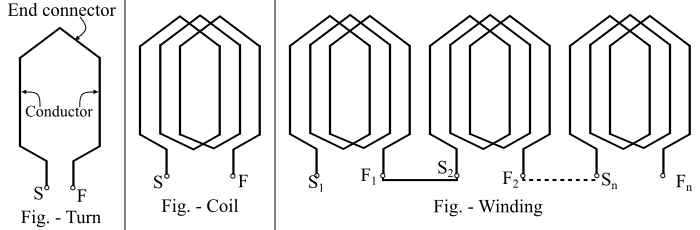# Basic Terms in Electrical Machine and their Definition

In this article, we will take a look at some of the basic terms used in Electrical Machines and their definition.

## Field Winding

The winding of the electrical machine through which a current is passed to produce the main flux is known as field winding.

## Armature Winding

The winding of the electrical machine in which voltage is induced by the action of electromagnetic induction is known as armature winding.

Some of the basic terms associated with the armature winding are as follows −

• Turn – When two conductors are connected to one end by an end connector, it is known as a turn.

• Coil – When several turns are connected in series, then it is called a coil.

• Winding – When several coils are connected in series, it is known as a winding.

The figure shows the turn, coil and winding. The beginning of the turn or coil is denoted by ‘S’ (Start) and the end of the turn or coil is denoted by ‘F’ (Finish).## Relationship between Electrical Degree and Mechanical Degree

The concept of electrical degrees is very useful in the study of electrical machines.

Let,

• $𝜃_{𝑚}$ = Mechanical degrees or angular measure in space

• $𝜃_{𝑒}$ = Electrical degrees or angular measure in cycles

Then, for an electrical machine having P-poles, the electrical degree is defined as follows −

$$\mathrm{𝜃_{𝑒} =\frac{𝑃}{2}𝜃_{𝑚} … (1)}$$

The advantage of the relation of eq. (1) is that expressions written in terms of electrical angles apply to electrical machines having any number of poles.

## Pole Pitch

The angular Distance between the centres of two adjacent poles on an electrical machine is known as pole pitch or pole span. Regardless of the number of poles in the electrical machine, a pole pitch is always 180° electrical.

$$\mathrm{One\:pole\:pitch =\frac{360° \:mechanical}{𝑃}= 180°\:electrical}$$

## Coil Pitch

As the two sides of a coil are placed in two slots on the stator core. The distance between the two sides of a coil is known as the coil pitch.

• If the coil pitch is equal to one pole pitch, the coil is known as the full pitch coil.

• When the coil pitch is less than one pole pitch, the coil is said to be short-pitch coil or fractional-pitch coil.